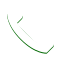# Venting InstallationsBalance is the key to having an efficient attic ventilation system. When balanced, a properly designed ventilation system will assure a continuous supply of air moves through the attic space, fighting off heat and moisture. Calculating the correct number of exhaust and intake vents is simple and will ensure you have an effective, balanced system.

The first step in determining how many vents you need is to calculate the Net Free Area (NFA) required. Most codes use the 1/300 rule for minimum residential attic ventilation recommendations. This means that for every 300 square feet of enclosed attic space, 1 square foot of ventilation is required – with half at the upper portion (exhaust vents) and half in the lower portion (intake vents). This formula is traditionally used for static roof vents which are rated for Net Free Area in terms of square inches.

Let’s go through an example… For a home with 2000 square feet of attic floor space, you’ll first divide 2000 by 300 (2000 / 300 = 6.66). You need 6.66 square feet of attic ventilation. Since you want a balanced system, you divide by 2 so that half of the ventilation is intake and half is exhaust. Thus, 6.66 divided by 2 = 3.33 square feet of attic ventilation for intake and 3.33 square feet of attic ventilation for exhaust. Because vents are rated in square inches, you need to convert the square feet required to square inches. This is accomplished by taking the square feet recommended and multiplying by 144. Thus, 3.33 X 144 = 480 square inches of attic ventilation is required for intake and 480 square inches for exhaust.

Once the recommended amount of Net Free Area is known and the type of vents have been selected, you can determine how many vents you will need. The next step is to divide the NFA required by the NFA rating of the vent. In our 2000 square feet example, we determined we needed 480 square inches for intake & 480 square inches for exhaust. For this example, let’s use the Lomanco 750 Slant Back Vent (50 square inches NFA) for the exhaust vents & the Deck-Air DA-4 (36 square inches NFA) for the intake vents. To calculate the number of 750 vents needed, divide 480 by 50 to get 9.6 vents. Rounding up, you would need ten (10) 750 Vents. Because you always want your intake NFA to meet or exceed the amount of exhaust NFA, we will take the amount of exhaust provided and divide by the NFA rating of the Deck-Air. To calculate the number of Deck-Air Vents needed, divide 500 (10 X 50) by 36 to get 13.9 vents. Thus, you would need 14 Deck-Air Vents.

If your head is now spinning from the calculations, don’t worry Lomanco has the tools needed to determine the number of vents needed (no calculator required!) Use the online ventilator calculator or download the Vent selector app and skip the math.CALL NOW FOR A FREE QUOTE